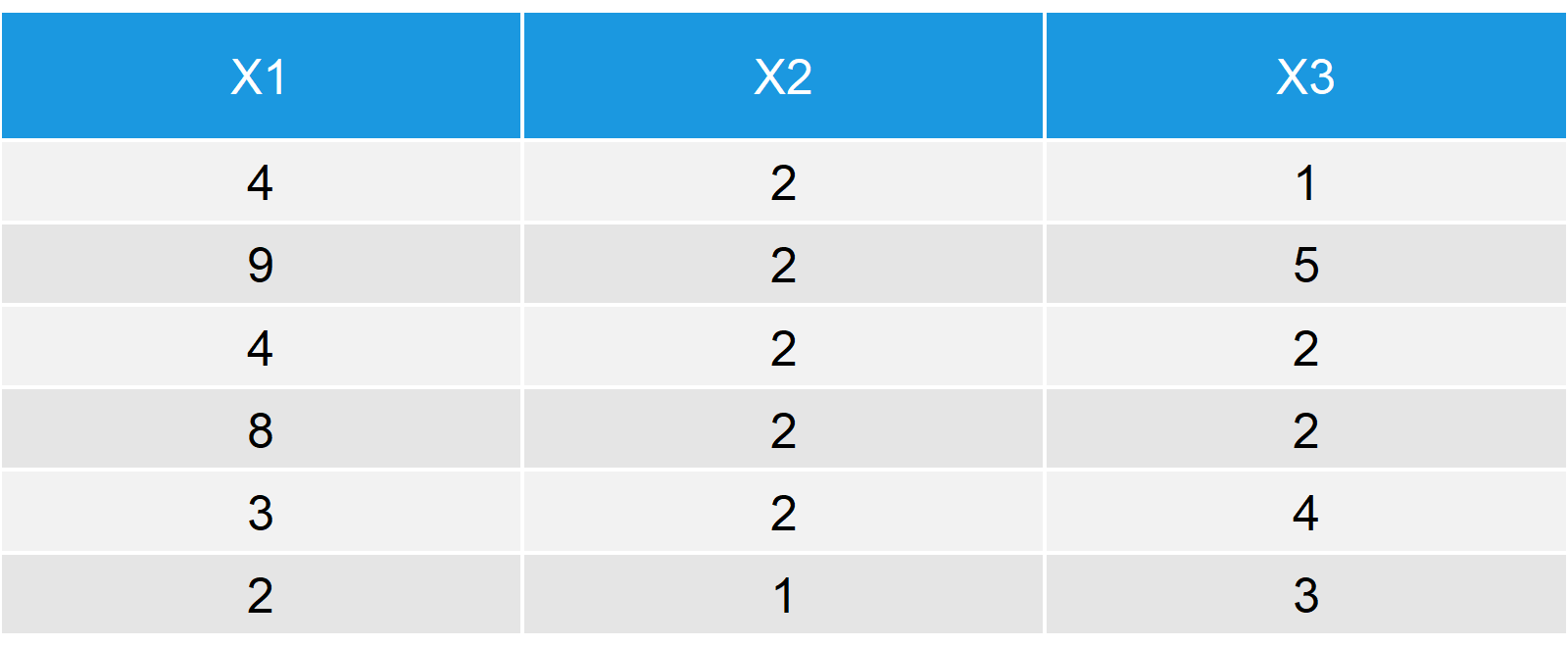# The ncol Function in R (3 Examples)

Basic R Syntax:

`ncol(data)`

The ncol R function returns the number of columns of a matrix or data frame. Above, you can find the command for the application of ncol in the R programming language.

You’d like to hear some more details? In the following tutorial, I’ll provide you with several examples of the usage of the ncol function in R.

## Example 1: Count Number of Columns of a Data Frame

Before we can dive into the application of the ncol command in R, let’s create an example data frame:

```set.seed(99999)                       # Seed for reproducibility
N <- 100                              # Sample size

x1 <- round(runif(N, 1, 10))          # Column 1
x2 <- round(runif(N, 0, 3))           # Column 2
x3 <- round(runif(N, 1, 5))           # Column 3

data_frame <- data.frame(x1, x2, x3)  # Data frame with 3 columnsTable 1: Example Data for the Application of the ncol R function.

As you can see based on Table 1, our data frame consists of 3 columns. Let’s check how we could investigate on that with the ncol function in R:

```ncol(data_frame)                      # Count the number of columns
# 3```

ncol returns the number 3 – seems correct!

## Example 2: Count the Number of Columns of a Matrix

The ncol function is easy to apply – also to matrices! Even if our data has the class matrix, we can apply the ncol command in the same manner.

First, let’s convert the data frame we used before into a matrix:

`mat <- as.matrix(data_frame)`

Then, let’s apply the ncol function:

```ncol(mat)
# 3```

Still 3 – very good.

## Example 3: ncol Returns NULL – A Common Mistake

A mistake that I see quite often is that people try to apply ncol to a vector (often, because they falsely think that their data is in data frame or matrix format).

The result is that R returns NULL, instead of the number of columns. Confusing…

I’ll illustrate that with some R code:

```set.seed(716253)                      # Set seed
vec1 <- rnorm(10, 5, 2)               # Some random data vector

ncol(vec1)                            # Apply the ncol R command
# NULL```

As you can see, the ncol command is not working for vectors. If you want to know the amount of values of a vector, you have to use the transpose function…

```ncol(t(vec1))                         # Transpose function in R
# 10```

…or even easier: the length function.

```length(vec1)                          # Length function in R
# 10```

## Video Examples: ncol and Similar R Functions in Practice

Do you need more examples? No problem! Have a look at the following YouTube video of the Statistics Globe YouTube channel.

Please accept YouTube cookies to play this video. By accepting you will be accessing content from YouTube, a service provided by an external third party.If you accept this notice, your choice will be saved and the page will refresh.